# First Midterm Exam l l November 07 2015

• Slides: 14
Download presentation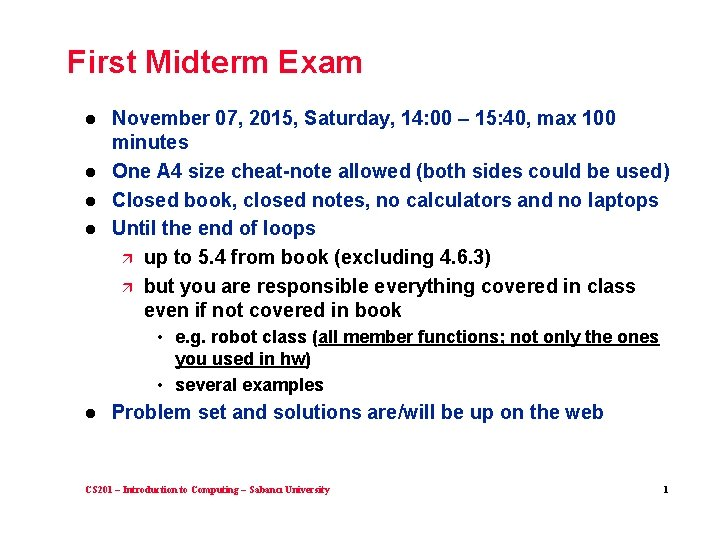First Midterm Exam l l November 07, 2015, Saturday, 14: 00 – 15: 40, max 100 minutes One A 4 size cheat-note allowed (both sides could be used) Closed book, closed notes, no calculators and no laptops Until the end of loops ä up to 5. 4 from book (excluding 4. 6. 3) ä but you are responsible everything covered in class even if not covered in book • e. g. robot class (all member functions; not only the ones you used in hw) • several examples l Problem set and solutions are/will be up on the web CS 201 – Introduction to Computing – Sabancı University 1First Midterm Exam – Exam Places if (lastname >= "Açılan" && lastname <= "Ezberli") cout << " FENS G 077 auditorium" << endl; else if (lastname >= "Fışkın" && lastname <= "Güzgeren") cout << " FASS G 022 " << endl; else if (lastname >= "Hammad" && lastname <= "Koçer") cout << " FASS G 049 " << endl; else if (lastname >= "Koçulu" && lastname <= "Özciğer") cout << " FASS G 052" << endl; else if (lastname >= "Özdamarlı" && lastname <= "Tekinel") cout << " FASS G 062 auditorium" << endl; else if (lastname >= "Telci" && lastname <= "Zor") cout << " FENS L 045" << endl; CS 201 – Introduction to Computing – Sabancı University 2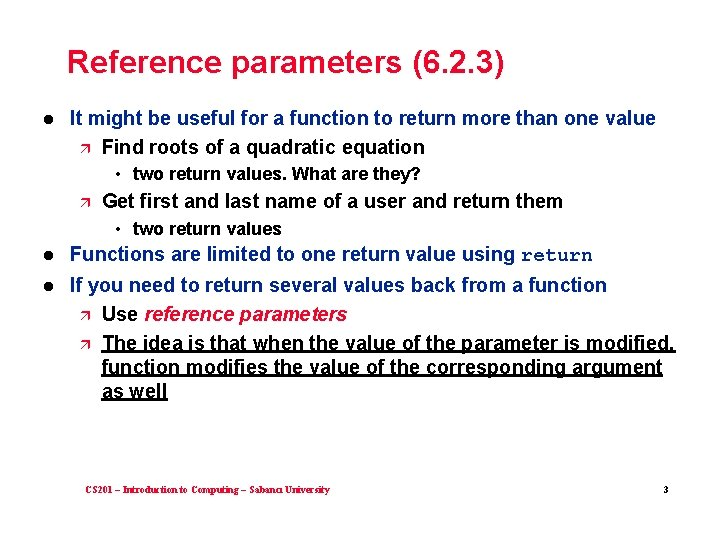Reference parameters (6. 2. 3) l It might be useful for a function to return more than one value ä Find roots of a quadratic equation • two return values. What are they? ä Get first and last name of a user and return them • two return values l Functions are limited to one return value using return l If you need to return several values back from a function ä Use reference parameters ä The idea is that when the value of the parameter is modified, function modifies the value of the corresponding argument as well CS 201 – Introduction to Computing – Sabancı University 3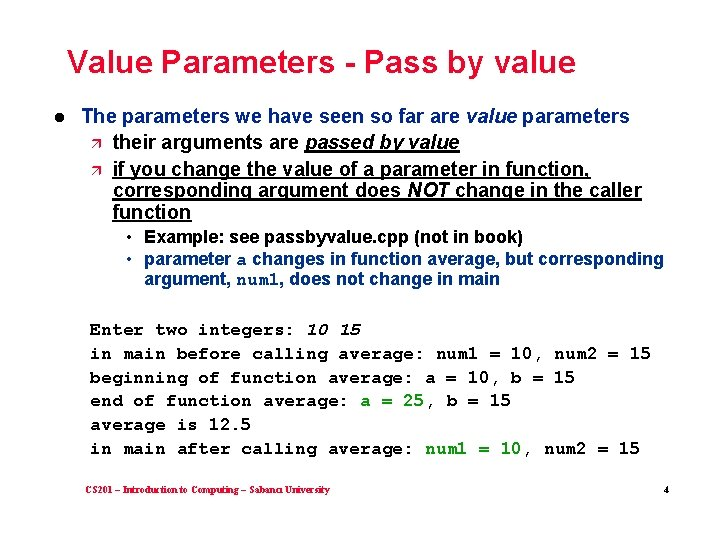Value Parameters - Pass by value l The parameters we have seen so far are value parameters ä their arguments are passed by value ä if you change the value of a parameter in function, corresponding argument does NOT change in the caller function • Example: see passbyvalue. cpp (not in book) • parameter a changes in function average, but corresponding argument, num 1, does not change in main Enter two integers: 10 15 in main before calling average: num 1 = 10, num 2 = 15 beginning of function average: a = 10, b = 15 end of function average: a = 25, b = 15 average is 12. 5 in main after calling average: num 1 = 10, num 2 = 15 CS 201 – Introduction to Computing – Sabancı University 4Reference Parameters – Pass by Reference l Reference parameters are used to change the value of the argument in the caller function as well ä ampersand between type and name void myfunction (int & num) ä ä if you change the value of a reference parameter in function, corresponding argument changes in the caller function Argument of a reference parameter must be a variable • This is a reasonable rule, because otherwise its value wouldn’t change ä See passbyreference. cpp (not in book) • parameter a changes in function average; corresponding argument, num 1, changes in main as well Enter two integers: 10 15 in main before calling average: num 1 = 10, num 2 = 15 beginning of function average: a = 10, b = 15 end of function average: a = 25, b = 15 average is 12. 5 in main after calling average: num 1 = 25, num 2 = 15 CS 201 – Introduction to Computing – Sabancı University 5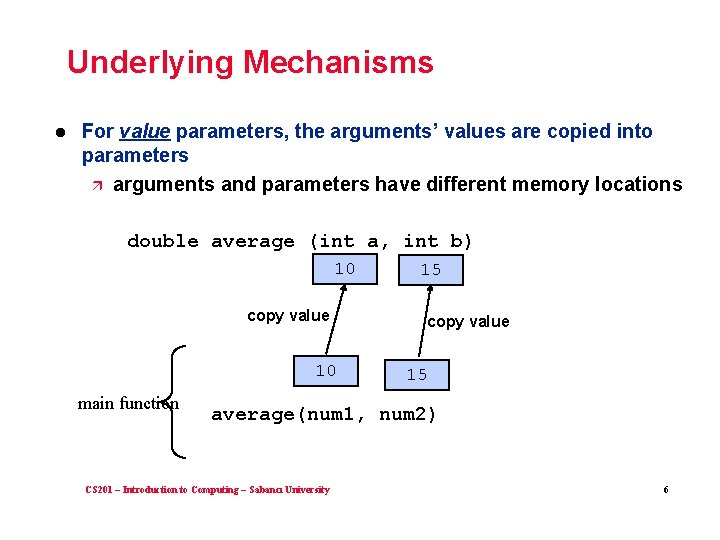Underlying Mechanisms l For value parameters, the arguments’ values are copied into parameters ä arguments and parameters have different memory locations double average (int a, int b) 10 copy value 10 15 copy value 15 main function average(num 1, num 2) CS 201 – Introduction to Computing – Sabancı University 6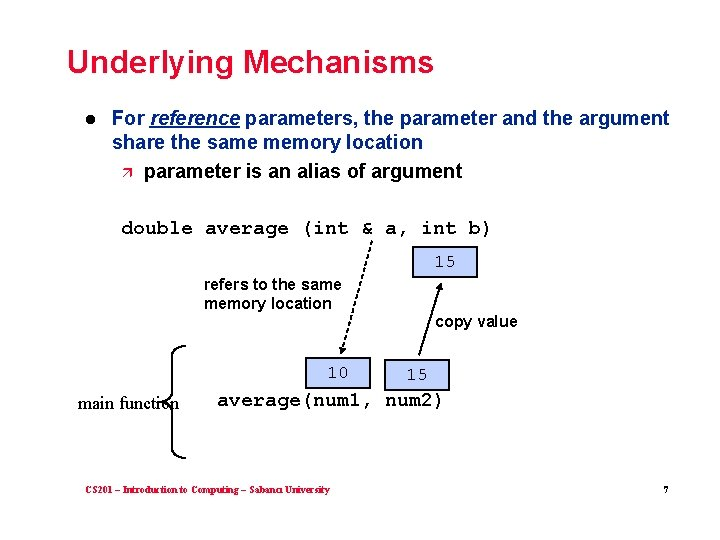Underlying Mechanisms l For reference parameters, the parameter and the argument share the same memory location ä parameter is an alias of argument double average (int & a, int b) 15 refers to the same memory location 10 copy value 15 average(num 1, num 2) main function CS 201 – Introduction to Computing – Sabancı University 7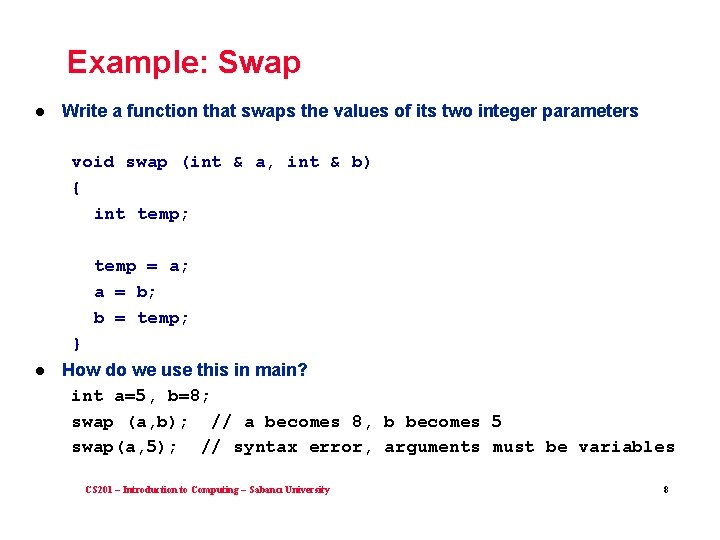Example: Swap l Write a function that swaps the values of its two integer parameters void swap (int & a, int & b) { int temp; temp = a; a = b; b = temp; } l How do we use this in main? int a=5, b=8; swap (a, b); // a becomes 8, b becomes 5 swap(a, 5); // syntax error, arguments must be variables CS 201 – Introduction to Computing – Sabancı University 8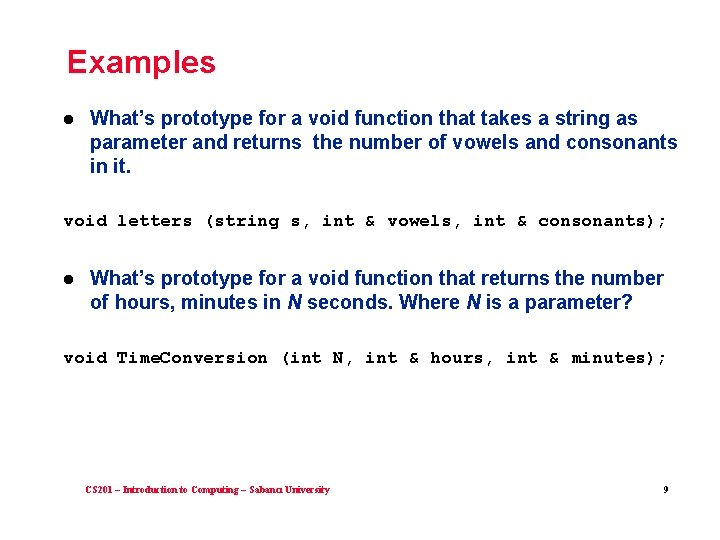Examples l What’s prototype for a void function that takes a string as parameter and returns the number of vowels and consonants in it. void letters (string s, int & vowels, int & consonants); l What’s prototype for a void function that returns the number of hours, minutes in N seconds. Where N is a parameter? void Time. Conversion (int N, int & hours, int & minutes); CS 201 – Introduction to Computing – Sabancı University 9Reference Parameters are not only to return multiple values l l Even if you have a single value to return, you may prefer to return it as a reference parameter, not as the return value of the function. For example: ä ä ä To. Lower function, defined in strutils, changes its argument to all lowercase. It is actually a void function, i. e. does not return anything as the function’s return value Prototype is void To. Lower(string & s); Example use string s = "He. LLo"; To. Lower(s); // s becomes "hello" CS 201 – Introduction to Computing – Sabancı University 10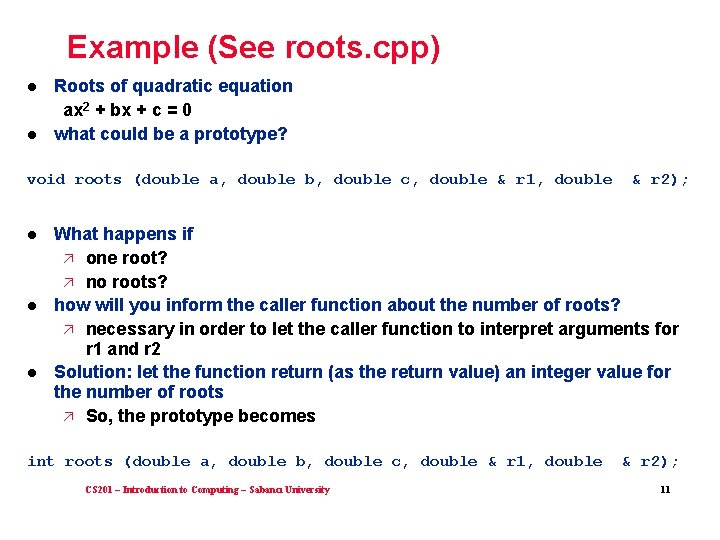Example (See roots. cpp) l l Roots of quadratic equation ax 2 + bx + c = 0 what could be a prototype? void roots (double a, double b, double c, double & r 1, double & r 2); l l l What happens if ä one root? ä no roots? how will you inform the caller function about the number of roots? ä necessary in order to let the caller function to interpret arguments for r 1 and r 2 Solution: let the function return (as the return value) an integer value for the number of roots ä So, the prototype becomes int roots (double a, double b, double c, double & r 1, double & r 2); CS 201 – Introduction to Computing – Sabancı University 11Parameter Passing: const-reference l Pass by value (value parameters) has overheads ä copying the value ä memory allocation for both parameter and argument l Sometimes we want to avoid the overhead of making the copy, but we don’t want to allow the argument to be changed. ä const-reference parameters avoid copies, but cannot be changed in the function • trying to change a const-reference parameter is a syntax error ä defined with const before a reference parameter void demo (const int & num, const string & s); CS 201 – Introduction to Computing – Sabancı University 12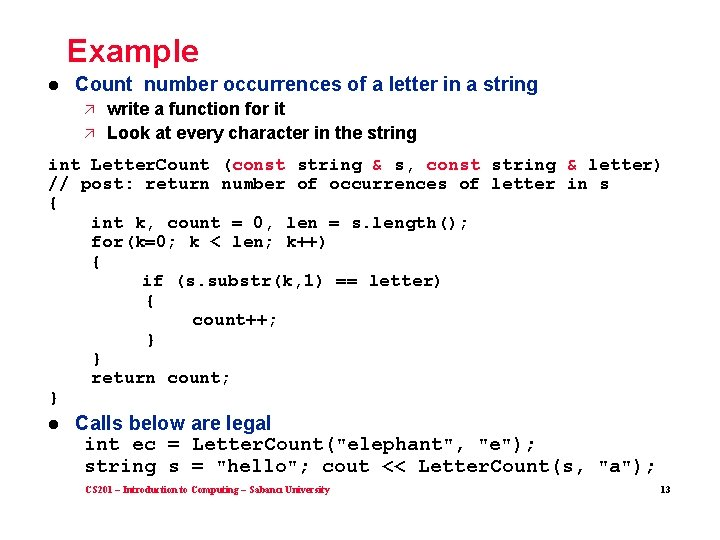Example l Count number occurrences of a letter in a string ä ä write a function for it Look at every character in the string int Letter. Count (const string & s, const string & letter) // post: return number of occurrences of letter in s { int k, count = 0, len = s. length(); for(k=0; k < len; k++) { if (s. substr(k, 1) == letter) { count++; } } return count; } l Calls below are legal int ec = Letter. Count("elephant", "e"); string s = "hello"; cout << Letter. Count(s, "a"); CS 201 – Introduction to Computing – Sabancı University 13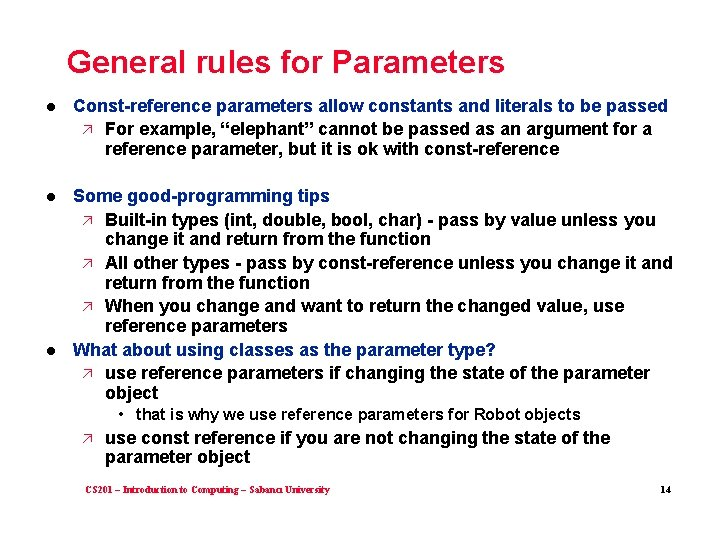General rules for Parameters l Const-reference parameters allow constants and literals to be passed ä For example, “elephant” cannot be passed as an argument for a reference parameter, but it is ok with const-reference l Some good-programming tips ä Built-in types (int, double, bool, char) - pass by value unless you change it and return from the function ä All other types - pass by const-reference unless you change it and return from the function ä When you change and want to return the changed value, use reference parameters What about using classes as the parameter type? ä use reference parameters if changing the state of the parameter object l • that is why we use reference parameters for Robot objects ä use const reference if you are not changing the state of the parameter object CS 201 – Introduction to Computing – Sabancı University 14